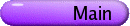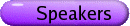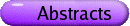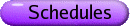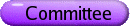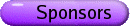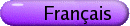Contributed Papers
Org: C. Robert Miers (Victoria)
[PDF]

LENNARD BAKKER, Brigham Young University
The Multiplier Group of a Quasiperiodic Flow
[PDF]

The multiplier group of an F-algebraic quasiperiodic flow on the n-torus is shown to be a finite index subgroup of the group of units in the ring of integers of the real algebraic number field F. It is also shown that the multiplier group of a quasiperiodic flow being {1,-1} implies the that the quasiperiodic flow is transcendental. The converses of these are validated for quasiperiodic flows on the 2-torus.

ROBB FRY, Thompson Rivers University, Kamloops, BC
Beyond partitions of unity
[PDF]

The tried and true method of uniformly approximating continuous functions by smooth functions on Banach spaces is through the use of smooth partitions of unity. Much effort over the last several decades has gone into establishing the existence of such smooth partitions of unity on ever wider classes of non-separable Banach spaces. One weakness of this technique is that it is difficult to arrange for the approximating function to have stronger properties in addition to Cp-Fréchet smoothness.

In this talk we shall first review the method of approximation via partitions of unity, and then discuss more recent approaches in which the smooth approximating function can be chosen also to be Lipschitz or possess a uniformly continuous derivative.

MUDASSAR IMRAN, Arizona State University, PO Box 871804, Tempe, AZ 85287-1804, USA
The Model of Antibiotic Resistance in Biofilm
[PDF]

A mathematical model of the effect of antibiotics on bacterial biofilm is considered. According to the US National Institute of Health, "Biofilms are medically important, accounting for over 80% of microbial infections in the body", such as otitis media, the most common acute ear infection in children in the US. Biofilms are highly resistant to antibiotics. Consequently, very high and/or long-term doses are often required to eradicate biofilm-related infections. We consider a two-compartments, chemostat-based model where one compartment has a very high dilution rate as compared to the other compartment. The high dilution rate compartment represents the fluid environment and the low dilution rate compartment represents the stagnant biofilm environment. A constant supply of nutrient and a periodically fluctuating antibiotic agent is supplied to the high dilution compartment. The model assumes that antibiotic increases the death rate as its concentration is increased. We use persistence as well as global bifurcation results for a mathematical analysis of periodic solutions. The model consists of a system of non-autonomous differential equations which govern the dynamics of the bacteria in biofilm.

This is joint work with Hal Smith.

IN-JAE KIM, University of Victoria
Smith Normal Form and Acyclic Matrices
[PDF]

An approach, based on the Smith Normal Form, is introduced to study the spectra of symmetric matrices with a given graph. The approach serves well to explain how the path cover number (resp. diameter of a tree T) is related to the maximum multiplicity occurring for an eigenvalue of a symmetric matrix whose graph is T (resp. the minimum number q(T) of distinct eigenvalues over the symmetric matrices whose graphs are T). The approach is also applied to a more general class of connected graphs G, not necessarily trees, in order to establish a lower bound on q(G).

MAIA LESOSKY, Guelph
Medical Imaging and Euclidean Motion Deconvolution
[PDF]

This talk will discuss the application of deconvolution methods to medical imaging data. The Radon transform forms the backbone for most computerized tomography (CT) imaging systems. Reconstruction of these images requires an implementation of the inverse Radon transform. There are a number of inversion algorithms available, however, most have serious deficiencies. This approach formulates the Radon transform as a convolution integral over the Euclidean motion group.

XIAO PING LIU, Department of Mathematics and Statistics, University of Regina, Regina, SK S4S 0A2
Determinantal inequalities for certain classes of totally nonnegative matrices
[PDF]

An n-by-n matrix A is called totally nonnegative, TN (totally positive, TP) if the determinant of every square submatrix (i.e., minor) of A is nonnegative (positive). A collection S of bounded ratios are said to be generators for all such bounded ratios if any bounded ratios can be written as a product ratios from this collection S. We define a particular class of totally nonnegative matrices, called STEP1, and establish all the generators for STEP1 matrices. All such generators have been shown to be bounded ratios for general TN matrices.

TUFAIL MALIK, Arizona State University, PO Box 871804, Tempe, AZ 85287-1804, USA
A Resource-Based Model of Microbial Quiescence
[PDF]

To analyze the ecological features of microbial quiescence, a model is proposed that involves "wake-up" rate and "sleep" rate at which the population transitions from a quiescent to an active state and back, respectively. These rates depend continuously on the resources and turn on and off at resource thresholds which may not coincide. The usual dichotomy is observed: the population is washed out under environmental stress and a single "survival" steady state exists otherwise. Proportional nutrient enrichment is used to explore analytically as well as numerically the nature of the steady state which bifurcates from the washout state.

This is joint work with Hal Smith.

MITJA MASTNAK, Dept. of Math, UBC, Room 121, 1984 Mathematics Road, Vancouver, BC V6T 1Z2
[PDF]

If L is an m-dimensional linear subspace of Mn×p, the space of n×p matrices, then we can identify the embedding km[( @ ) || (® )] L Í Mn×p with a bilinear map km ×kn ® kp or with a linear map kmÄkn® kp. If we "switch" km and kn then we get an embedding kn®Mm×o, and hence an n-dimensional linear subspace L¢ of Mm×o. We study the relationship between L and L¢ and give examples of situations where this duality can be exploited.

MICHAEL SZAFRON, Department of Mathematics and Statistics, University of Saskatchewan, Saskatoon, SK
The Probability of Knotting after a Local Strand Passage in an Unknotted SAP
[PDF]

Due to DNA's structure, it is prone to several different topological entanglement problems. The cell must be able to resolve each of these problems because the problems interfere with vital cell functions. For instance, knotted DNA cannot replicate successfully. The cell therefore must have some mechanism by which the DNA can be unknotted. This mechanism is the interaction of the DNA with the topoisomerase enzymes.

The topoisomerase enzymes interact locally with the DNA and pass one strand of DNA through itself via the enzyme-bridged transient break in the DNA [RW94]. Since these local strand-passages can potentially change the knot-type of the DNA [DSKC85], [WC91], experimentalists can use the frequency of knots produced to characterize topoisomerase action on DNA topology [WC86].

It is of interest whether these local strand-passages are implemented at random locations in the DNA. In order to investigate this problem, a simplified model of an unknotted ring polymer was implemented via Monte Carlo simulation to estimate the probability that a ring polymer has knot-type K after a local strand passage has occurred within the ring polymer. The model, some estimates of the knotting probabilities, and an observation will be presented.

LUIS VERDE-STAR, Universidad Autonoma Metropolitana, Mexico City
Computation of the matrix exponential via the dynamic solution
[PDF]

Let A be a square matrix with characteristic (or minimal) polynomial w(x), of degree n+1. The dynamical solution g(t) associated with w is the solution of the homogeneous differential equation w(D)y(t) = 0 that has the initial values Dk g(0) = 0 for 0 £ k < n and Dn g(0) = 1.

The matrix exponential is given by

 etA = nå k=0 Dk g(t) wn-k(t),
where the wj are the Horner polynomials associated with w. We explore some analytic and numerical aspects of the use of the above formula, and try to explain the sources of the main computational problems. Other functions of matrices can be computed by analogous formulas. See L. Verde-Star, Functions of matrices, Linear Alg. Appl. 406(2005), 285-300.